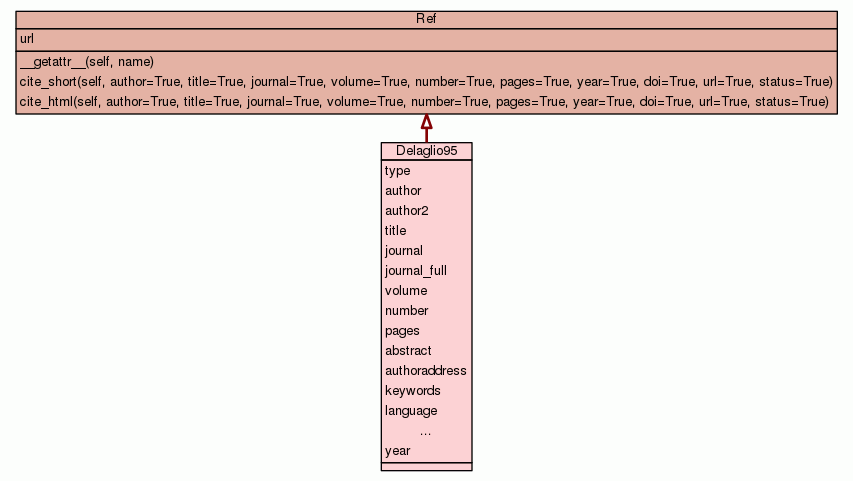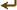[frames] | no frames]

# Class Delaglio95

source codeBibliography container.

 Instance Methods

Inherited from `Ref`: `__getattr__`, `cite_html`, `cite_short`

 Class Variables
type = `'journal'`
hash(x)
author = `'Delaglio, F., Grzesiek, S., Vuister, G.W., Zhu, G., ...`
hash(x)
author2 = `[['Frank', 'Delaglio', 'F.', None], ['Stephan', 'Grz...`
hash(x)
title = `'NMRPipe: a multidimensional spectral processing syste...`
hash(x)
journal = `'J. Biomol. NMR'`
hash(x)
journal_full = `'Journal of Biomolecular NMR'`
hash(x)
volume = `'6'`
hash(x)
number = `'3'`
hash(x)
pages = `'277-293'`
hash(x)
abstract = `'The NMRPipe system is a UNIX software environment ...`
authoraddress = `'Laboratory of Chemical Physics, National Inst...`
keywords = `'Magnetic Resonance Spectroscopy/*instrumentation ;...`
language = `'eng'`
doi = `'10.1007/BF00197809'`
hash(x)
pubmed_id = `8520220`
hash(x)
status = `'published'`
hash(x)
year = `1995`
hash(x)

Inherited from `Ref`: `url`

 Class Variable Details

### author

hash(x)

Value:
 ````'``Delaglio, F., Grzesiek, S., Vuister, G.W., Zhu, G., Pfeifer, J. and B``ax, A.``'` ```

### author2

hash(x)

Value:
 ````[``[``'``Frank``'``, ``'``Delaglio``'``, ``'``F.``'``, `None`]``,` `[``'``Stephan``'``, ``'``Grzesiek``'``, ``'``S.``'``, `None`]``,` `[``'``Geerten``'``, ``'``Vuister``'``, ``'``G.``'``, ``'``W.``'``]``,` `[``'``Guang``'``, ``'``Zhu``'``, ``'``G.``'``, `None`]``,` `[``'``John``'``, ``'``Pfeifer``'``, ``'``J.``'``, `None`]``,` `[``'``Ad``'``, ``'``Bax``'``, ``'``A.``'``, `None`]``]` ```

### title

hash(x)

Value:
 ````'``NMRPipe: a multidimensional spectral processing system based on UNIX ``pipes.``'` ```

### abstract

Value:
 ````'``The NMRPipe system is a UNIX software environment of processing, grap``hics, and analysis tools designed to meet current routine and research``-oriented multidimensional processing requirements, and to anticipate ``and accommodate future demands and developments. The system is based o``n UNIX pipes, which allow programs running simultaneously to exchange ``streams of data under user control. In an NMRPipe processing scheme, a`` stream of spectral data flows through a pipeline of processing progra``ms, each of which performs one component of the overall scheme, such a``...` ```

 ````'``Laboratory of Chemical Physics, National Institute of Diabetes and Di``gestive and Kidney Diseases, National Institutes of Health, Bethesda, ``MD 20892, USA.``'` ```
 ````'``Magnetic Resonance Spectroscopy/*instrumentation ; *Software``'` ```